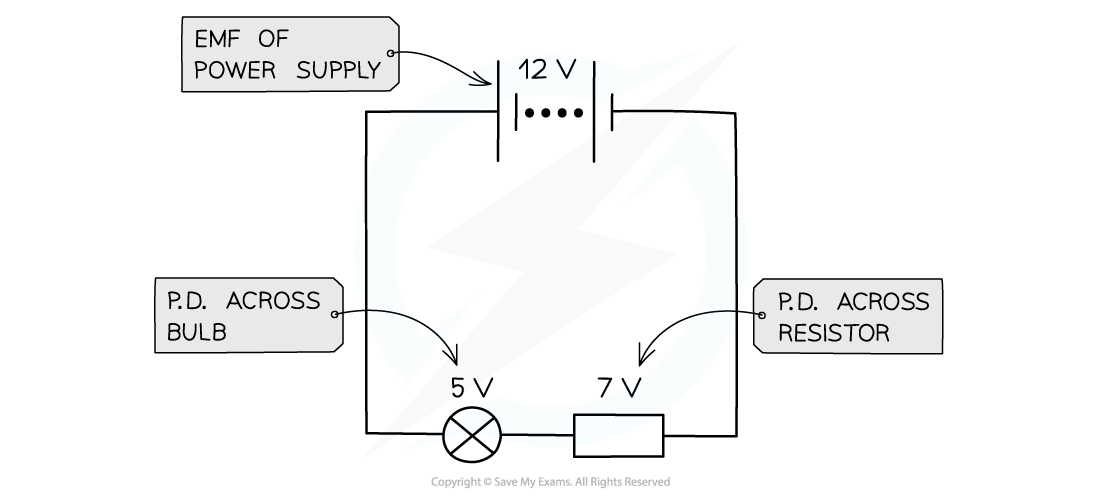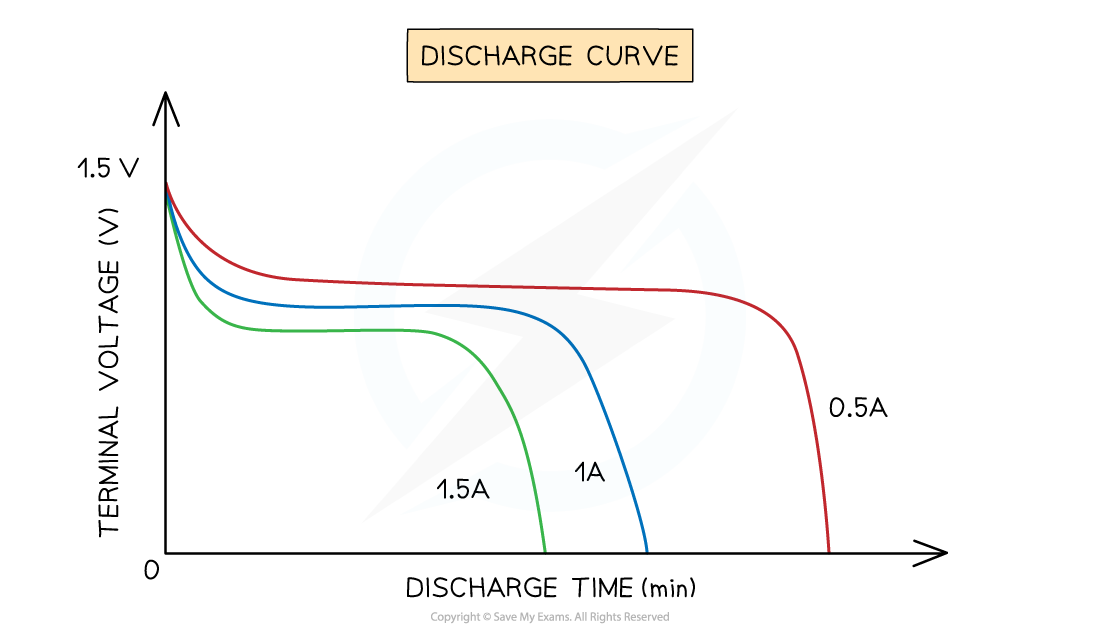# IB DP Physics: HL复习笔记5.3.3 Terminal Potential Difference

### Terminal Potential Difference

#### Defining Potential Difference

• A cell makes one end of the circuit positive and the other negative. This sets up a potential difference V across the circuit
• The potential difference across a component in a circuit is defined as the energy transferred per unit charge flowing from one point to another
• The energy transfer is from electrical energy into other forms
• Potential difference is measured in volts (V). This is the same as a Joule per coulomb (J C-1)
• If a bulb has a voltage of 3 V, every coulomb of charge passing through the bulb will transfer 3 J of energy
• The potential difference of a power supply connected in series is always shared between all the components in the circuitThe potential difference is the voltage across each component in a circuit

#### Calculating Potential Difference

• The potential difference is defined as the energy transferred per unit charge
• Another measure of energy transfer is work done
• Therefore, potential difference can also be defined as the work done per unit chargePotential difference is the work done per unit charge

#### Terminal Potential Differnce & Lost Volts

• The terminal potential difference (p.d) is the potential difference across the terminals of a cell
• If there was no internal resistance, the terminal p.d would be equal to the e.m.f
• It is defined as:

V = IR

• Where:
• V = terminal p.d (V)
• I = current (A)
• R = load resistance (Ω)
• If a cell has internal resistance r, the terminal p.d is always lower than the e.m.f
• If you have a load resistor R across the cell's terminals, then the terminal p.d V is equal to the p.d across the load resistor
• In a closed circuit, current flows through a cell and a potential difference develops across the internal resistance
• Since resistance opposes current, this reduces the energy per unit charge (voltage) available to the rest of the external circuit
• This difference is called the ‘lost volts’
• Lost volts is usually represented by little v
• It is defined as the voltage lost in the cell due to internal resistance
• So, from conservation of energy, we can say:

v = e.m.f − terminal p.d

v = ε – V = Ir (Ohm’s law)

• Where:
• v = lost volts (V)
• I = current (A)
• r = internal resistance of the battery (Ω)
• ε = e.m.f (V)
• V = terminal p.d (V)
• Therefore, lost volts is the difference between the e.m.f and the terminal p.d

#### Discharging a Cell

• When a cell is discharging, it will not discharge a constant amount of voltage
• Instead, an initial high amount that slowly decreases over time is discharged ending in a rapid decrease
• This means that cells make a distinctive discharge curve with a drop, plateau and final rapid dropTypical discharge curves for a 1.5 V terminal cell showing discharge for a 0.5A, 1A and 1.5A drawing current

#### The Capacity of a Cell

• The capacity of a cell is the amount of charge that it contains and is able to discharge
• This is measured in Ampere hours (A hr)
• When a cell has a certain capacity the amount of current drawn from this cell will impact the amount of time that it can run for
• In the image above three different drawing currents are shown for the same 1.5 V cells
• The relationship between current drawn and hours of cell lifetime is a simple linear relationship
• As an example: A 100 A.hr capacity battery is able to provide 100 hours of 1A current
• However, the same battery when fully charged can give 50 hours of charge for a 2A current or 25 hours for a 4A current

#### Worked Example

A lamp is connected to a 240 V mains supply and another to a 12 V car battery.Both lamps have the same current, yet 240 V lamp glows more brightly. Explain in terms of energy transfer why the 240 V lamp is brighter than the 12 V lamp.• Both lamps have the same current, which means charge flows at the same rate in both
• The 240 V lamp has 20 times more voltage than the 12 V lamp
• Voltage is the energy transferred (work done) per unit charge
• This means the energy transferred to each coulomb of charge in the 240 V lamp is 20 times greater than for the 12 V lamp
• This makes the 240 V lamp shine much brighter than the 12 V lamp

#### Exam Tip

• Think of potential difference as being the energy per coulomb of charge transferred between two points in a circuit
• If the exam question states 'a battery of negligible internal resistance', this assumes that e.m.f of the battery is equal to its voltage. Internal resistance calculations will not be needed here.
• If the battery in the circuit diagram includes internal resistance, then the e.m.f equations must be used.